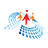Post
R
Learn R programming• ### R for Advanced Analytics - AI42 -S02 Ep. 01

Oct 18, 2021 2.3k
• ### Plotting Charts In R

Sep 13, 2021 3.7k
• ### Linear Regression In R

Sep 06, 2021 3.4k
• ### Introduction To Machine Learning Using R

Aug 19, 2021 3.4k
• ### Introduction To R

May 20, 2021 5.4k
• ### Data Wrangling And Visualization In R

May 18, 2021 4k
• ### Introduction to R (Session 2) - AI42 Ep.9

May 06, 2021 3.4k
• ### Introduction to R (Session 1) - AI42 Ep.8

Apr 23, 2021 4.4k
• ### How To Perform Stratified Sampling On Dataset In R

Feb 26, 2021 24.4k
• ### How To Create Samples Of Dataset In R

Feb 22, 2021 25.3k
• ### How To Use Simple Random Sampling Technique To Create Samples Of Dataset In R

Feb 22, 2021 8.9k
• ### How To Create Subsets Of Data Using Logical Values In R

Feb 17, 2021 2.8k
• ### How To Create Subsets Using Negative Numerical Data In R

Feb 12, 2021 4.7k
• ### How To Create Subsets Using Positive Numbers In R

Feb 09, 2021 3.1k
• ### How To Create A Blank Subset Of Data In R

Feb 08, 2021 2.8k
• ### How To Evaluate Variables On The Basis Of Dependent Variables In R

Jan 29, 2021 3.5k
• ### How To Calculate The Mode Of Variables In R

Jan 28, 2021 19k
• ### How To Implement Models Together With Variables And Dependencies In R

Jan 28, 2021 2.9k
• ### How To Calculate The Median Of Variables In R

Jan 25, 2021 21.5k
• ### How To Calculate The Mean Of Variables In R

Jan 22, 2021 80.7k
• ### How To Represent Variables As Function For Model In R

Jan 21, 2021 3.3k
• ### How To Insert Multiple Variables For A Model In R

Jan 21, 2021 5.2k
• ### How To Remove Unnecessary Variables For A Model In R

Jan 20, 2021 10.6k
• ### How To Evaluate Dependency Among Variables In R

Jan 20, 2021 6.2k
• ### How To Use Comparison Operators In R

Jan 04, 2021 2.9k